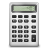### TCP/IP Subnet CalculatorHere's a free, handy utility that you can use to calculate the properties of an IP subnet.  Given an IP Address and a subnet mask, we can calculate the network address, the first and last addresses in the range of addresses that are valid for that subnet, and the broadcast address.

This utility is written in JavaScript, so it runs entirely in your browser.  If you'd like to learn about how the calculations work, you may be interested to look at my PowerShell version, complete with a discussion of the bit math.  See Calculating TCP/IP Subnets with PowerShell.

Here's the JavaScript version.  Just enter an IP address and a subnet mask, and the code will do the calculations:

IP Subnet Calculator